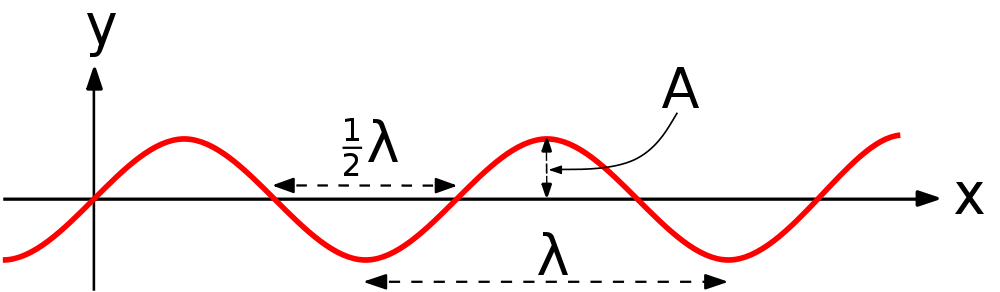# Waves

A wave is a disturbance or vibration that transmits energy, but not matter.## Wave Terms

Medium = material that the waves travel through

sound waves are vibrations of air

ocean waves are ripples in water

Crest = high point on a wave

Trough = low point on a wave

Wavelength (λ) = distance traveled by a single wave

Amplitude (A) = maximum displacement from rest

See the Pen wave properties by lilgreenland (@lilgreenland) on CodePen.

## The Wave Equation

v = velocity (m/s)

• The velocity is determined by the medium.
• λ = wavelength (m)

f = frequency (Hz, 1/s) = cycles per seconds

T = Period (s) = time for one cycle

See the Pen waves by lilgreenland (@lilgreenland) on CodePen.

Example: Playing middle C on a piano produces a sound with a frequency of 256 Hz. What is the period of the sound wave?

T = 1/f
T = 1/256 s

Example: An air horn sounds at a frequency of 220 Hz. If the speed of sound in air is 330 m/s what is the wavelength of the sound wave?

v = λf
λ = v/f
λ = 330/220
λ = 1.5m

Example: The distance between successive crests in a series of water waves is 4.0 m, and the crests travel 8.6 m in 5.0 s. Calculate the frequency of a block of wood bobbing up and down on these water waves.

λ = 4.0m
v = 8.6m/5.0s = 1.72m/s
v = λ f
f = v / λ
f = 1.72 / 4.0
f = 0.43 Hz